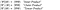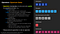# Qubit, An Intuition #2 — Inner Product, Outer Product, and Tensor Product in Bra-ket Notation

The beauty of Quantum Mechanics for Quantum Computation, featuring IBM Quantum

`TL;DR; 2 qubits inner product, outer product, and tensor product in bra-ket notation, with examples.Please refer to the previous article (published in July 12 2021), “Qubit, An Intuition #1 — First Baby Steps in Exploring the Quantum World” for a discussion on a single qubit as a computing unit for quantum computation.`

the first article, “Qubit, An Intuition #1 — First Baby Steps in Exploring the Quantum World,” we discussed the intuition of a quantum bit (qubit) as a computing unit in a Quantum computer, in comparison to a binary digit (bit) in a Classical computer. We also discussed Bra-ket notation, Bloch sphere, along with the hybrid classical-quantum approach.

In this follow-on article, we will discuss the inner product, outer product, and tensor product. These are some of the basic mathematical foundations to understand quantum computation.

# Quantum Bit

Indeed, a single quantum bit (qubit) is exciting with its nature of being in superposition. Hence |Ψ> = α|0> + β|1>. The quantum state Psi (|Ψ>) is in the linear combination of |0> and |1> with the probability of being in state |0> is |α|², and the probability of being in state|1> is |β|².

α and β are the probability amplitudes while α* and β* are the complex conjugates of α and β, respectively. It fulfills the normalization constraint, such that |α|²+|β|²=1 or α*α+β*β=1, where α, β ∈ ℂ².

# Inner Product, Outer Product, and Tensor Product

However, we need more qubits to do meaningful quantum computation. Let’s say that we have the following two qubits, Psi|Ψ> and Phi |Φ>. Each has its respected quantum state.

Note that the relationship for bra and ket for a quantum state. The bra is a complex conjugate transpose of ket and vice versa.

We can have the following valid operations for|Ψ> and |Φ>. We call the operation between bra <Ψ| and ket |Φ>as the inner product, ket |Ψ>and bra <Φ|as the outer product, and ket |Ψ> and ket |Φ> as the tensor product.Bra-ket notation for two-qubit operations: inner product, outer product, and tensor product.

However, the following operation is invalid:

Let’s give a value for α, β, γ, and δ (note that α, β, γ, δ ∈ ℂ²). By assigning α=-4/5i, β=3/5, γ=1/2, and δ=sqrt(3)/2 for example, we can have some values to work on for ket |Ψ>, bra <Ψ|, ket |Φ>, and bra <Φ|.

For the first qubit |Ψ> with α=-4i/5 and β=3/5, we find the ket |Ψ> and bra <Ψ|. First, we do a qubit validity test by verifying that |α|²+|β|²=1.

Then, for the second qubit |Φ> with γ=1/2, and δ=sqrt(3)/2, we find the ket |Φ> and bra <Φ|. First, we do a qubit validity test by verifying |γ|²+|δ|²=1.

We can then illustrate the two-qubit operations for the inner product, outer product, and tensor product.

## Inner Product — <ΨΦ>

A product of two quantum states bra Psi <Ψ| and ket Phi |Φ> is called an inner product, producing a value. An inner product is also called an overlap, the overlap between quantum states.

## Outer Product — |ΨΦ|

A product of two quantum states, ket Psi|Ψ> and bra Phi <Φ|, is called an outer product, producing a matrix. An outer product is also called a projection.

## Tensor Product — |ΨΦ>

A product of two quantum states, ket Psi|Ψ> and ket Phi |Φ> is called a tensor product, producing a column vector with length 2ⁿ (where n is the number of qubits).

If we square all individual vector elements and sum them up, the total must be 1.

It means that the following normalization constraint applies:

|αγ|², |αδ|², |βγ|², and |βδ|² represent the probability of measuring 00, 01, 10, and 11, respectively — which is 16%, 48%, 9%, and 27%.

The following illustration describes the tensor product further:

We use the tensor product in a quantum circuit for combining multiple qubits (two or more qubits) with quantum operators to transform qubits from one state to another, therefore performing quantum computation.An example of quantum gates: NOT (flip gate), CNOT (Controlled-NOT), H (Hadamard), and Z (phase flip gate). Quantum gates are used to perform quantum computation in a quantum circuit.

# Moving Forward

We can do a full or partial measurement of qubits. We will discuss this further in the next article, “Qubit, An Intuition #3 — Quantum Measurement, Full and Partial Qubits.”